Question

# A circular rod 1.60 nm in diameter and 44.0 meters in length has resistivity 34.0 nano-Ohms...

A circular rod 1.60 nm in diameter and 44.0 meters in length has resistivity 34.0 nano-Ohms meters.

What is the length-wise resistance of this rod, in Ohms?

Given:

Length of the rod,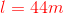Diameter of the rod,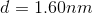Radius,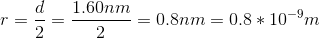Area of cross-section,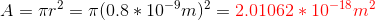Resistivity,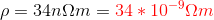==============================

Resistance,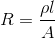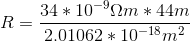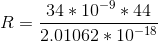ANSWER: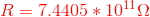==============================

#### Earn Coins

Coins can be redeemed for fabulous gifts.

Similar Homework Help Questions
• ### A wire of length 0.47 meters and cross-section area 7.1 cm 2 has resistivity 96x10 -6...

A wire of length 0.47 meters and cross-section area 7.1 cm 2 has resistivity 96x10 -6 Ohm-meters. What is the resistance (in Ohms) of this wire?

• ### A Si wire of diameter 2μm and length 200 micrometers has a resistance of 1000 ohms....

A Si wire of diameter 2μm and length 200 micrometers has a resistance of 1000 ohms. Determine the resistivity of that Si in terms of ohm-cm. Suppose you make the same wire using copper, what should be its resistance?

• ### A cylindrical wire has a resistance r and resistivity p if its length and diameter are...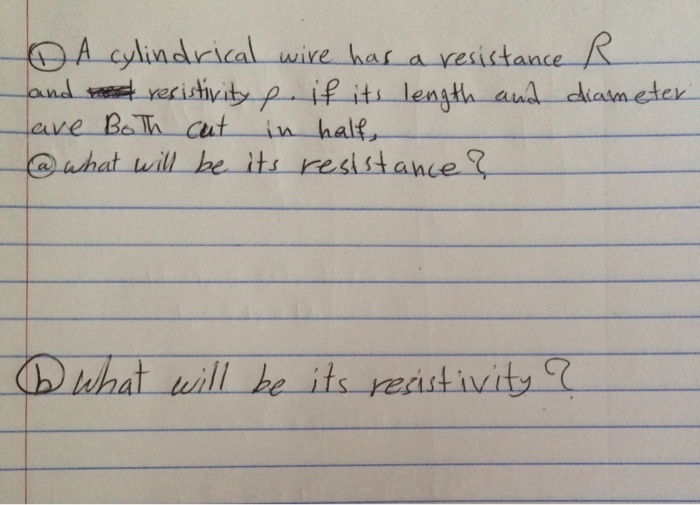A cylindrical wire has a resistance r and resistivity p if its length and diameter are both cut in half what will be its resistance? What will be its resistivity?

• ### A 16.50 ?? long wire has a diameter of 0.355 ?? and a resistivity of7.65 ?...

A 16.50 ?? long wire has a diameter of 0.355 ?? and a resistivity of7.65 ? 10,- Ω ??. ( a ) Calculate its resistance (b) If a 3.85 ???? battery is connected to the wire, what current exists in the circuit? I changed those three values to meters as below, what do I do next please Length = 0.165 m Diameter = 0.000355 m Resistivity = 7.65 * 10^-9 Ω m

• ### A wire whose resistivity, p=4.92 x 10-8 Ωm has a length L = 26 m and a diameter of d = 6.1 x 10-4 m.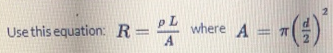A wire whose resistivity, p=4.92 x 10-8 Ωm has a length L = 26 m and a diameter of d = 6.1 x 10-4 m. What is the resistance of this wire in ohms, a? Please round your answer to one decimal place.

• ### A circular rod has a diameter of 10 cm, length of 10 m, and a modulus...A circular rod has a diameter of 10 cm, length of 10 m, and a modulus of elasticity of 30 MPa. Find the stiffness of the rod in N/m. k= 23562 N/m k= 94248 N/m k= 16233 N/m k= 235.62 *106 N/m

• ### A certain laboratory experiment requires an aluminum wire of length of 34.0 m and a resistance...

A certain laboratory experiment requires an aluminum wire of length of 34.0 m and a resistance of 2.60 Ω at 20.0°C. What diameter wire must be used? (The resistivity of aluminum at 20.0°C is 2.82 ✕ 10−8 Ω · m.) ______ mm

• ### Consider a wire with resistivity of 9.2 x10-7 Ω m, a diameter of 4.5 mm, and...

Consider a wire with resistivity of 9.2 x10-7 Ω m, a diameter of 4.5 mm, and a length of 33 m. What is the resistance of the wire in ohms (Ω).

• ### Consider a wire 1.00 meters in length with 0.100 mm diameter and resistivity, ? = 1.18             ...

Consider a wire 1.00 meters in length with 0.100 mm diameter and resistivity, ? = 1.18              x              10’(Ωm. Give all calculated answers to 3 significant figures and report voltages in millivolts. a) (10 pts) Calculate the resistance of the wire. ?)*+, = ________________________ b) (10 pts) The same wire is placed in an AC voltage divider circuit with a function generator. It follows, in series, a resistor, R=5.00 kΩ. An oscilloscope’s channel one shows a 9.10 peak-to-peak voltage sine wave...

• ### .A cylindrical metal rod has a resistance R. A second rod that is made of the...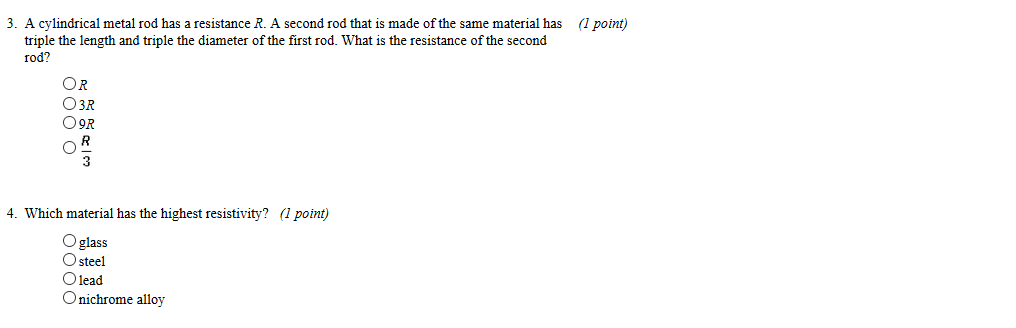.A cylindrical metal rod has a resistance R. A second rod that is made of the same material has (1 potnt) triple the length and triple the diameter of the first rod. What is the resistance of the second rod? OR O3R O9R 4. Which material has the highest resistivity? (l point) O glass O steel Olead Onichrome alloy